# Quick Tip: Implementing the Bubble Sort in AS3In this Quick Tip, I'll show you how and why the Bubble Sort algorithm works, and how to implement it in AS3. You'll end up with a class that you can use in any Flash project, for sorting any array.

## Final Result Preview

Here's a simple demo of the result of the bubble sort algorithm:

Of course, this SWF doesn't prove much on its own! Grab the source files and you can edit the input array yourself.

## Step 1: Creating the BubbleSort Class

As this algorithm will be used more than once, it's a good idea to create a class for it, so that we can easily use it in any AS3 project:

Set up a basic Flash project, and inside the project folder create a file BubbleSort.as. (We will create a tester file here too, so we can test it.)

If you dont know how to work with classes please check this tutorial: How to Use a Document Class in Flash.

We wont need the constructor, so get rid of it! Your class should look like this:

## Step 2: How the Algorithm Works

This algorithm is not the fastest or most efficient method of sorting a series of numbers, but it is the easiest one to understand.

This image sums it up; at every stage, each pair of numbers are compared, starting from the end, and swapped (by means of a "spare" temp variable) if they are in the wrong order.

Once all consecutive pairs have been checked, this guarantees that the number at the start is the largest number in the sequence; we then repeat, checking every pair of numbers apart from the number at the start. Once all consecutive pairs have been checked, we know that the first two numbers in the sequence are in the correct order (they are the largest and second-largest). We keep going until we've put every number in the correct order.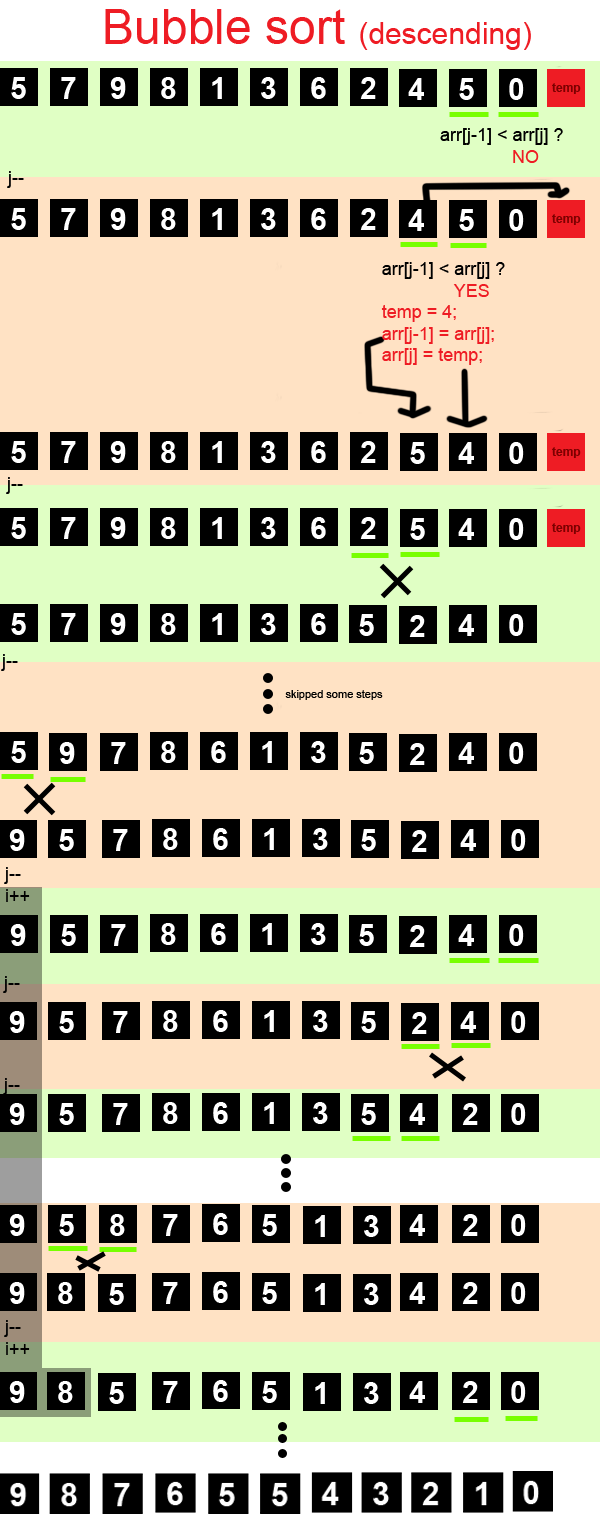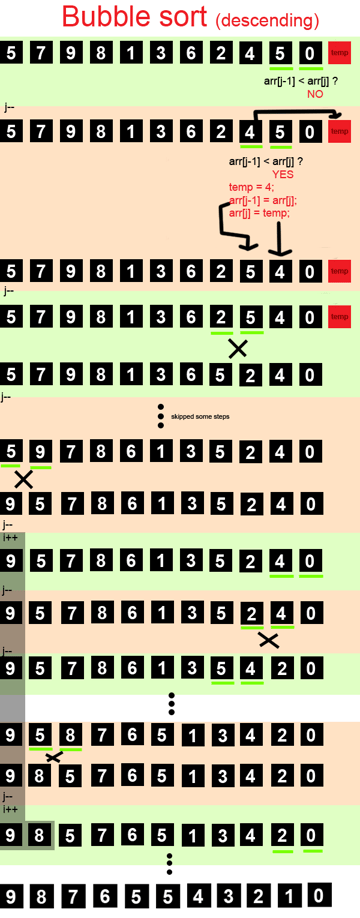It's called the "bubble sort" because, on each pass through the array, the biggest number "floats" to the top of the array, like a bubble in water.

Lets start writing the code. We'll call the main function bsort():

The function gets two parameters. The first parameter, arr, will be the array to be sorted; the second paramter, sortType will be used to decide if the user wants the array to be sorted in ascending or descending order.

In the function we declare a temp variable which will hold the elements of the array in case we need to swap the two elements. You may wonder why it is not a Number. It's because our class will be able to handle String arrays too, sorting them alphabetically; we can convert numbers to strings and back again, but we can't convert strings to numbers and back again, so we use a String for this variable, just to be safe.

We use an if-else block to split our code into two branches, depending on which direction the user wants to sort in. (If the user does not provide a valid choice, the program will fire an error.)

The difference between the code in either branch will be just one character: either < or >.

As you can see, we're using nested for loops. One goes from the first element to the last element of the array; the other goes backwards.

Let's inspect the inner "j" loop first. As the earlier diagram shows, we begin by comparing the last two elements of the array, which are arr[j-1] and arr[j] (in the first iteration). If arr[j-1] is less than arr[j] they need to be swapped.

In either case we subtract one from j (through the "j--" call in line 131), which changes which pair of numbers will be compared on the next loop.

j starts at a value of arr.length-1, and ends with a value of 1, meaning that the inner for loop checks every consecutive pair, starting with the last pair (where j equals arr.length-1) and ending with the first pair (where j equals 1).

Now let's look at the outer "i" loop. Once all the pairs have been checked and swapped as necessary, i is increased (through the "i++" call in line 129). This means that, the next time round, j will start at arr.length-1 again, but end at 2 this time - meaning that the first pair in the sequence will not be checked or swapped. This is exactly what we want, since we know that the first number is in the correct position.

As it goes on, eventually there will be only two elements that need to be checked in the inner loop. Once they're done, we know we've sorted the array!

Here's what that looks like in code:

Now we can use the same logic to create the ascending sort:

We need only to change the comparison operator in the if block of the inner loop:

## Step 3: Creating the Tester Application

Create a new flash file, tester.fla, in the same folder as BubbleSort.as. Create two dynamic text fields, name one input_arr and the other one output_arr.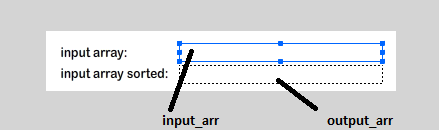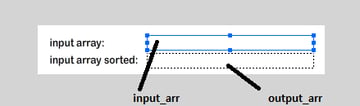After creating the appearance, we must create and link the document class.

Create a file Tester.as and link this to tester.fla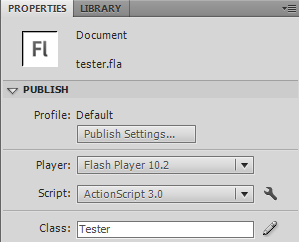Now we can finally use our class in the Tester.as:

In this line, we call the bsort() function of our variable bs (which is an instance of BubbleSort):

This function returns an array, so we can assign this as the new value of our original input array.

Save everything and try your work out.

## Conclusion

In this tutorial we created a function to help us sort an Array. We could improve the efficiency; for more on that, you can read Wikipedia - Bubble Sort

If you really wish to see how fast this algorithm is compared to the other options (like quicksort), take a look at sorting-algorithms.com.